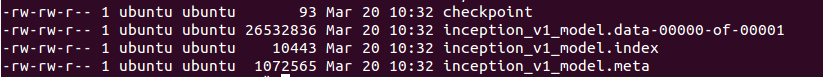# Tensorflow之训练模型保存与加载实例

1. TensorFlow模型是什么样的？
2. 如何保存TensorFlow模型？
3. 如何恢复预测/转移学习的TensorFlow模型？
4. 如何使用导入的预先训练的模型进行微调和修改?

# 1. 什么是TensorFlow模型？

a) Meta graph：
这是一个协议缓冲区，它保存了完整的Tensorflow图形;即所有变量、操作、集合等。该文件以.meta作为扩展名。

b) Checkpoint file：

这是一个二进制文件，它包含了所有的权重、偏差、梯度和其他所有变量的值。这个文件有一个扩展名.ckpt。然而，Tensorflow从0.11版本中改变了这一点。现在，我们有两个文件，而不是单个.ckpt文件:


1. mymodel.data-00000-of-00001

2. mymodel.index

.data文件是包含我们训练变量的文件，我们待会将会使用它。1. inception_v1.meta

2. inception_v1.ckpt

3. checkpoint

# 2. 保存TensorFlow模型

saver = tf.train.Saver()

saver.save(sess, 'my-test-model')

import tensorflow as tf

w1 = tf.Variable(tf.random_normal(shape=), name='w1')

w2 = tf.Variable(tf.random_normal(shape=), name='w2')

saver = tf.train.Saver()

sess = tf.Session()

sess.run(tf.global_variables_initializer())

saver.save(sess, 'my_test_model')

# This will save following files in Tensorflow v >= 0.11

# my_test_model.data-00000-of-00001

# my_test_model.index

# my_test_model.meta

# checkpoint

saver.save(sess, 'my_test_model',global_step=1000)


1. my_test_model-1000.index

2. my_test_model-1000.meta

3. my_test_model-1000.data-00000-of-00001

4. checkpoint

saver.save(sess, 'my-model', global_step=step,write_meta_graph=False)

#saves a model every 2 hours and maximum 4 latest models are saved.

saver = tf.train.Saver(max_to_keep=4, keep_checkpoint_every_n_hours=2)

import tensorflow as tf

w1 = tf.Variable(tf.random_normal(shape=), name='w1')

w2 = tf.Variable(tf.random_normal(shape=), name='w2')

saver = tf.train.Saver([w1,w2])

sess = tf.Session()

sess.run(tf.global_variables_initializer())

saver.save(sess, 'my_test_model',global_step=1000)

# 3. 导入训练好的模型

a)创建网络

saver = tf.train.import_meta_graph('my_test_model-1000.meta')

b)载入参数

with tf.Session() as sess:

new_saver = tf.train.import_meta_graph('my_test_model-1000.meta')

new_saver.restore(sess, tf.train.latest_checkpoint('./'))

with tf.Session() as sess:

saver = tf.train.import_meta_graph('my-model-1000.meta')

saver.restore(sess,tf.train.latest_checkpoint('./'))

print(sess.run('w1:0'))

##Model has been restored. Above statement will print the saved value of w1

# 4.使用导入的模型

import tensorflow as tf

#Prepare to feed input, i.e. feed_dict and placeholders

w1 = tf.placeholder("float", name="w1")

w2 = tf.placeholder("float", name="w2")

b1= tf.Variable(2.0,name="bias")

feed_dict ={w1:4,w2:8}

#Define a test operation that we will restore

w4 = tf.multiply(w3,b1,name="op_to_restore")

sess = tf.Session()

sess.run(tf.global_variables_initializer())

#Create a saver object which will save all the variables

saver = tf.train.Saver()

#Run the operation by feeding input

print sess.run(w4,feed_dict)

#Prints 24 which is sum of (w1+w2)*b1

#Now, save the graph

saver.save(sess, 'my_test_model',global_step=1000)

#How to access saved variable/Tensor/placeholders

w1 = graph.get_tensor_by_name("w1:0")

## How to access saved operation

op_to_restore = graph.get_tensor_by_name("op_to_restore:0")

import tensorflow as tf

sess=tf.Session()

#First let's load meta graph and restore weights

saver = tf.train.import_meta_graph('my_test_model-1000.meta')

saver.restore(sess,tf.train.latest_checkpoint('./'))

# Now, let's access and create placeholders variables and

# create feed-dict to feed new data

graph = tf.get_default_graph()

w1 = graph.get_tensor_by_name("w1:0")

w2 = graph.get_tensor_by_name("w2:0")

feed_dict ={w1:13.0,w2:17.0}

#Now, access the op that you want to run.

op_to_restore = graph.get_tensor_by_name("op_to_restore:0")

print sess.run(op_to_restore,feed_dict)

#This will print 60 which is calculated

#using new values of w1 and w2 and saved value of b1. 

import tensorflow as tf

sess=tf.Session()

#First let's load meta graph and restore weights

saver = tf.train.import_meta_graph('my_test_model-1000.meta')

saver.restore(sess,tf.train.latest_checkpoint('./'))

# Now, let's access and create placeholders variables and

# create feed-dict to feed new data

graph = tf.get_default_graph()

w1 = graph.get_tensor_by_name("w1:0")

w2 = graph.get_tensor_by_name("w2:0")

feed_dict ={w1:13.0,w2:17.0}

#Now, access the op that you want to run.

op_to_restore = graph.get_tensor_by_name("op_to_restore:0")

#Add more to the current graph

#This will print 120.

......

......

saver = tf.train.import_meta_graph('vgg.meta')

# Access the graph

graph = tf.get_default_graph()

## Prepare the feed_dict for feeding data for fine-tuning

#Access the appropriate output for fine-tuning

fc7= graph.get_tensor_by_name('fc7:0')

#use this if you only want to change gradients of the last layer

fc7 = tf.stop_gradient(fc7) # It's an identity function

fc7_shape= fc7.get_shape().as_list()

new_outputs=2

weights = tf.Variable(tf.truncated_normal([fc7_shape, num_outputs], stddev=0.05))

biases = tf.Variable(tf.constant(0.05, shape=[num_outputs]))

output = tf.matmul(fc7, weights) + biases

pred = tf.nn.softmax(output)

# Now, you run this with fine-tuning data in sess.run()

04-18904609-281万+
11-108万+
01-179498
11-011296
10-2557
05-156068
01-125万+